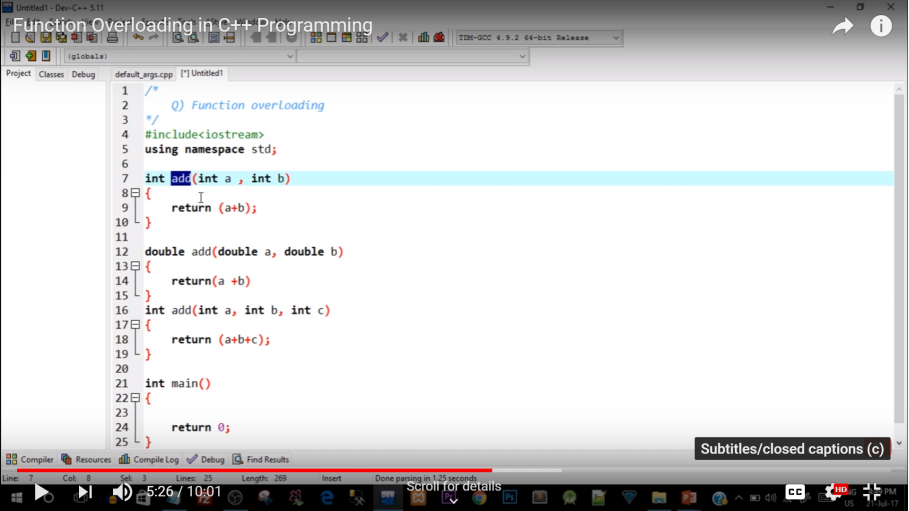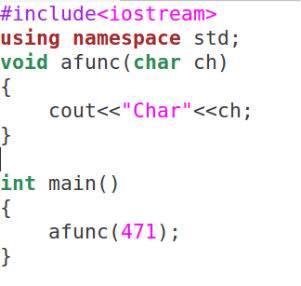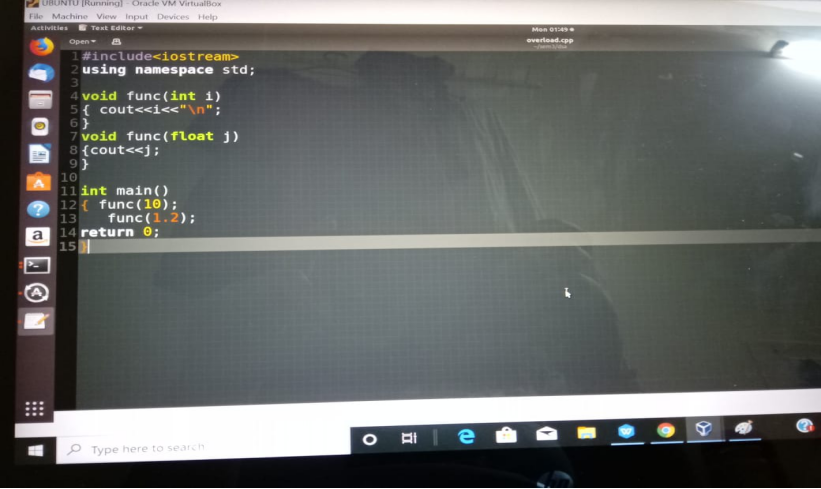INTRODUCTION:-

OVERLOADING MEANS ASSIGNING MULTIPLE MEANINGS TO A FUNCTION NAME OR OPERATOR SYMBOL.

IT ALLOWS MULTIPLE DEFINITIONS OF FUNCTIONS WITH SAME NAME BUT DIFFERENT SIGNATURES.

C++ SUPPORTS-

FUNCTION OVERLOADING CAN BE CONSIDERED AS AN EXAMPLE OF POLYMORPHISM FEATURE IN C++.

IT ALLOWS FUNCTIONS THAT CONCEPTUALLY PERFORM THE SAME TASK ON OBJECTS OF DIFFERENT TYPES TO BE GIVEN THE SAME NAME.

• DIFFERENT TYPES OF PARAMETERS
• DIFFERENT NUMBER OF PARAMETERS
• DIFFERENT SEQUENCE OF PARAMETERS

FOR EG:-

void print();

void print(int a);

void print(float a);

void print(int a,int b);

void print(int a,double b);

void print(double a,int b);STEPS INVOLVED IN FINDING BEST MATCH FOR A FUNCTION CALL:-

A call to an overloaded function is resolved to a particular instance of the function, there are three possible cases, a function call may result in:

• One match  - A match is found for the function call.
• No match - No match is found for the function call.
• Ambiguous Match - More than one defined instance for the function call.

EXACT MATCH:-

For example, there are two functions with same name "afunc":

void afunc(int);

void afunc(double);

The function call afunc(10); is matched to void afunc(int); and compiler invokes corresponding function definition as 10 is of type int.

A MATCH THROUGH PROMOTION:-

If no exact match is found, an attempt is made to achieve a match through promotion of the actual argument.

Recall that the conversion of integer types (char, short, int) into int - integral promotion.

For example, consider the following code fragment:

void afunc (int);

void afunc (float);

afunc('c'); Will invoke afunc(int) AND Compiler resolves square('c') to square(int).

A MATCH THROUGH APPLICATION OF STANDARD C++ RULES:-

If no exact match or match through a promotion is found, an attempt is made to achieve a match through a standard conversion of the actual argument. Consider the following example,'int'  argument is converted to char.

CAUSES OF AMBIGUITY:-

TYPE CONVERSION-OUTPUT:-